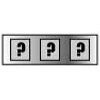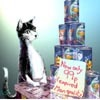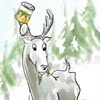#### You may also like### Forgotten Number

I have forgotten the number of the combination of the lock on my briefcase. I did have a method for remembering it...### Human Food

Sam displays cans in 3 triangular stacks. With the same number he could make one large triangular stack or stack them all in a square based pyramid. How many cans are there how were they arranged?### Sam Again

Here is a collection of puzzles about Sam's shop sent in by club members. Perhaps you can make up more puzzles, find formulas or find general methods.

# Triangle Numbers

### Why do this problem?

This problem allows students to investigate the relationship between triangle numbers expressed as a sum and triangle numbers expressed visually. Students may focus on either representation to arrive at a conclusion. The problem can be concrete with students focusing on small numbers or can be extended to give a general result.

### Possible approach

This printable worksheet may be useful: Triangle Numbers .

You could discuss the structure of triangle numbers as a sum (i.e. add $2$, then $3$, then $4$, then $5$, etc.) and then ask students to describe the visual layout of the triangle numbers coloured in the grid. Students can then experiment on paper to try to spot patterns from which a general conjecture might be made.

It can be helpful to consider this problem as a process by which we move from one triangle number to the next. What changes with each step? Is this different for odd and even numbers?

### Key questions

• What can you see in the grid?
• Where would you expect the next pair of triangle numbers to go? Were you correct?
• Can you describe how to move from one triangle number to the next?
• What does moving one square down the multiplication grid do to the number?
• What is the effect of moving across by one column in the grid?

### Possible extension

• Can you create a general algebraic expression for the $n$th triangle number?

### Possible support

Providing grids for students to draw on might make the connections clearer.
Ask them to compare consecutive triangle numbers and explore the differences.